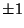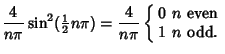## Square WaveThe square wave is a periodic waveform consisting of instantaneous transitions between two levels which can be denoted. The square wave is sometimes also called the Rademacher Function. Let the square wave have period. The square wave function is Odd, so the Fourier Series hasandThe Fourier Series for the square wave is therefore